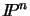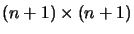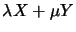Next: Projective Stereo vision Up: A Hierarchy of Geometries Previous: From Affine to Euclidean

# Summary

Projective spaceis invariant under the n(n+2)parameter projective group ofmatrices up to scale (8 dof in 2D, 15 dof in 3D). The fundamental projective invariant is the cross ratio which requires four objects in a projective pencil (one parameter configuration, or homogeneous linear combination of two basis objects). Projective space has notions of subspace incidence and an elegant duality between points and hyperplanes, but no notion of rigidity, distance, points at infinity', or sidedness/betweenness. It is the natural arena for perspective images and uncalibrated visual reconstruction.

N-dimensional affine space is invariant under the n(n+1)parameter affine group of translations and linear deformations (rotations, non-isotropic scalings and skewings). Affine space is obtained from projective space by fixing an arbitrary hyperplane to serve as the hyperplane of points at infinity': requiring that this be fixed puts n constraints on the allowable projective transformations, reducing them to the affine subgroup. The fundamental affine invariant is ratio of lengths along a line. Given 3 aligned points A,B,C, the length ratio AB/AC is given by the cross ratio A,B;D,C, where D is the line's affine point at infinity. Affine space has notions of `at infinity', sidedness/betweenness, and parallelism (lines meeting at infinity), but no notion of rigidity, angle or absolute length.

Similarity or scaled Euclidean space is invariant under the n(n+1)/2+1 parameter similarity group of rigid motions (rotations and translations) and uniform scalings. The fundamental similarity invariants are angles and arbitrary length ratios (including non-aligned configurations). Euclidean space is obtained from affine space by designating a conic in the hyperplane at infinity to serve as the absolute conic. The similarity group consists of affine transformations that leave the n(n+1)/2-1 parameters of this conic fixed. Angles can be expressed using the cross ratio and properties of this conic. Scaled Euclidean space has all the familiar properties of conventional 3D space, except that there is no notion of scale or absolute length.

Fixing this final scale leaves us with the n(n+1)/2 parameter Euclidean group of rigid motions of standard Euclidean space.Next: Projective Stereo vision Up: A Hierarchy of Geometries Previous: From Affine to Euclidean
Bill Triggs
1998-11-13